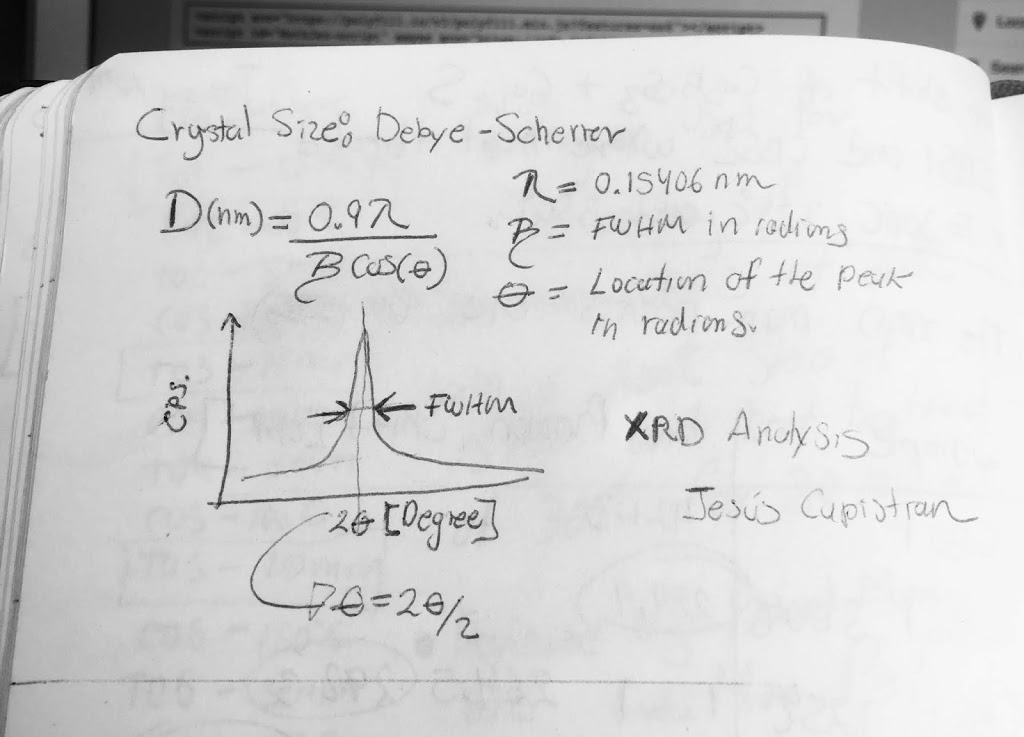• Post author:

This tip is useful for engineering and science bloggers who want to share their knowledge on the web. Here is the way I found. I recently tested it on the Blogger system,  the purpose is to share science tutorials with you using the correct math way.

Let’s do it!

<script src="https://polyfill.io/v3/polyfill.min.js?features=es6"></script>
<script id="MathJax-script" async src="https://cdn.jsdelivr.net/npm/mathjax@3/es5/tex-mml-chtml.js"></script>

Step 2: Write code in a new post following using latex language inside:

• Inline:           $$latex code here$$
• Paragraph:    $latex code here$

Step 3: Equation test: Debye -Scherrer  for crystal size calculation

[latex display=”true”] D(\text{nm}) = \frac{0.9\lambda}{\beta cos{\theta}}[/latex]
• D: Crystal size in nanometers
• $\lambda$: 0.15406 nm  X-Ray wavelength
• $\beta$:  FWHM in radians (Full width at half maximum)
• $\theta$: Angle location of the peak in radians.

If you have any questions, please comment on the post.### Jesus Capistran

Developing thin-film solar cells

### This Post Has 13 Comments

1.Jose Darias

not working in my posts. how did you do it? thanks a lot

1.I think you should add the backslash: \
Sorry for my mistake. I’ll fix it right now.

Example:

Step 3: Equation test: Debye -Scherrer for crystal size calculation

$D(\text{nm}) = \frac{0.9 \lambda}{\beta\cos{\theta}}$

D: Crystal size
$$\lambda$$ : 0.15406 nm X-Ray wavelength
$$\beta$$ : FWHM in radians (Full width at half maximum)
$$\theta$$ : Angle location of the peak in radians.

1.Jose Darias

Well done my friend!! It works fine now for me!!! Thansk a lot and don’t worry because we have a lot of time to learn a lot of things right now!!! Isaa Newton was in quarantine when he wrote all the major theorems about Calculus and Newtonian Phisycs due to the bubonic plague. Can you suggest some LaTeX guide to start formatting some text or if you have one please to send me to my email. Thanks a lot.

2.Jose Darias

By the way, some symbols. like Integrals got cutted when show in the post when rendered. Can you please show me how to fix that problem??

1.You can try display mode: $$\int_{a}^{b} x^2 dx$$. I test it on blogger and work it.

1.You can copy the following code:

Integral $$\int_{a}^{b} x^2 dx$$ inside text

$\int_{a}^{b} x^2 dx$

1.Jose Darias

Hello again Jesus:

I tried all the options you described in the comments above but Im still having the same issue; integrals do not show properly on my blog. They look like they show from half up but half bottom is always hide.

1.Hi Jose,

Could you try some examples from: https://www.mathjax.org/#samples ?

I test it on my old blogspot: https://capistran.blogspot.com/2020/01/how-to-add-math-on-your-blog-mathjax.html

Note: I can not figure what is your problem. I was thinking about your internet browser but I read this plugin supports the major and update actual web browser.

3.Hey Jesus I think actually is a bug with some rendering settings in Version 3 so I just moved to version 2 and everything worked fine .I really don’t know much about settings and programming only basic things like HTML and a few other simple settings. I had to overcome the problem with old version until Mathjax v3 post a new release. Thanks a lot! Keep in touch!! Take care of COVID19!!

4.Anupam

I have been trying for the longest time to make this work but it just doesn’t seem to work for me. This is what i have in the html

We can introduce a constant of proportionality called the decay rate constant $\lambda$ whose value depends on the type of radioactive substance we have.
([-\frac{dN}{N}=\lambda dt])

And it comes off as

We can introduce a constant of proportionality called the decay rate constant $\lambda$ whose value depends on the type of radioactive substance we have. ([-\frac{dN}{N}=\lambda dt]) ( [-\frac{dN}{N}=\lambda dt] )

Any help would be greatly appreciated because I have been looking all over the place to make equations appear in my blog.

1.Inline:           \ ( latex code here \ )
Paragraph:    \ [ latex code here \ ]

Note: Don’t leave a space between \ and ( .
$-\frac{dN}{N}=\lambda dt$

Note 2: See more examples in my Blogger site: https://capistran.blogspot.com/2020/01/how-to-add-math-on-your-blog-mathjax.html

5.Gracias fue mas facil asi. ya tengo la forma como agregar estas formulas y hacer mis post

1.Que bueno que este post te ayudo a configurar tu espacio. Compartenos tu blog !!

Saludos desde Mexico